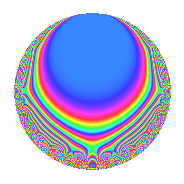# Properties

 Label 11.4.cLevel 11 Weight 4 Character orbit c Rep. character $$\chi_{11}(3,\cdot)$$ Character field $$\Q(\zeta_{5})$$ Dimension 8 Newforms 1 Sturm bound 4 Trace bound 0

# Related objects

## Defining parameters

 Level: $$N$$ = $$11$$ Weight: $$k$$ = $$4$$ Character orbit: $$[\chi]$$ = 11.c (of order $$5$$ and degree $$4$$) Character conductor: $$\operatorname{cond}(\chi)$$ = $$11$$ Character field: $$\Q(\zeta_{5})$$ Newforms: $$1$$ Sturm bound: $$4$$ Trace bound: $$0$$

## Dimensions

The following table gives the dimensions of various subspaces of $$M_{4}(11, [\chi])$$.

Total New Old
Modular forms 16 16 0
Cusp forms 8 8 0
Eisenstein series 8 8 0

## Trace form

 $$8q$$ $$\mathstrut -\mathstrut 7q^{2}$$ $$\mathstrut -\mathstrut 3q^{3}$$ $$\mathstrut +\mathstrut 3q^{4}$$ $$\mathstrut -\mathstrut 7q^{5}$$ $$\mathstrut -\mathstrut 29q^{6}$$ $$\mathstrut -\mathstrut 35q^{7}$$ $$\mathstrut +\mathstrut 47q^{8}$$ $$\mathstrut +\mathstrut 31q^{9}$$ $$\mathstrut +\mathstrut O(q^{10})$$ $$8q$$ $$\mathstrut -\mathstrut 7q^{2}$$ $$\mathstrut -\mathstrut 3q^{3}$$ $$\mathstrut +\mathstrut 3q^{4}$$ $$\mathstrut -\mathstrut 7q^{5}$$ $$\mathstrut -\mathstrut 29q^{6}$$ $$\mathstrut -\mathstrut 35q^{7}$$ $$\mathstrut +\mathstrut 47q^{8}$$ $$\mathstrut +\mathstrut 31q^{9}$$ $$\mathstrut +\mathstrut 40q^{10}$$ $$\mathstrut +\mathstrut 67q^{11}$$ $$\mathstrut +\mathstrut 190q^{12}$$ $$\mathstrut -\mathstrut 65q^{13}$$ $$\mathstrut -\mathstrut 196q^{14}$$ $$\mathstrut -\mathstrut 121q^{15}$$ $$\mathstrut -\mathstrut 377q^{16}$$ $$\mathstrut -\mathstrut 31q^{17}$$ $$\mathstrut -\mathstrut 102q^{18}$$ $$\mathstrut +\mathstrut 148q^{19}$$ $$\mathstrut +\mathstrut 342q^{20}$$ $$\mathstrut +\mathstrut 334q^{21}$$ $$\mathstrut +\mathstrut 647q^{22}$$ $$\mathstrut -\mathstrut 12q^{23}$$ $$\mathstrut -\mathstrut 447q^{24}$$ $$\mathstrut -\mathstrut 201q^{25}$$ $$\mathstrut -\mathstrut 140q^{26}$$ $$\mathstrut +\mathstrut 72q^{27}$$ $$\mathstrut -\mathstrut 42q^{28}$$ $$\mathstrut -\mathstrut 199q^{29}$$ $$\mathstrut -\mathstrut 114q^{30}$$ $$\mathstrut -\mathstrut 361q^{31}$$ $$\mathstrut +\mathstrut 324q^{32}$$ $$\mathstrut -\mathstrut 232q^{33}$$ $$\mathstrut -\mathstrut 298q^{34}$$ $$\mathstrut +\mathstrut 237q^{35}$$ $$\mathstrut +\mathstrut 120q^{36}$$ $$\mathstrut +\mathstrut 81q^{37}$$ $$\mathstrut -\mathstrut 52q^{38}$$ $$\mathstrut +\mathstrut 365q^{39}$$ $$\mathstrut +\mathstrut 532q^{40}$$ $$\mathstrut -\mathstrut 31q^{41}$$ $$\mathstrut +\mathstrut 170q^{42}$$ $$\mathstrut -\mathstrut 650q^{43}$$ $$\mathstrut -\mathstrut 1208q^{44}$$ $$\mathstrut +\mathstrut 452q^{45}$$ $$\mathstrut +\mathstrut 1204q^{46}$$ $$\mathstrut +\mathstrut 857q^{47}$$ $$\mathstrut +\mathstrut 644q^{48}$$ $$\mathstrut +\mathstrut 1375q^{49}$$ $$\mathstrut -\mathstrut 147q^{50}$$ $$\mathstrut -\mathstrut 246q^{51}$$ $$\mathstrut -\mathstrut 590q^{52}$$ $$\mathstrut -\mathstrut 1493q^{53}$$ $$\mathstrut -\mathstrut 3100q^{54}$$ $$\mathstrut -\mathstrut 1583q^{55}$$ $$\mathstrut -\mathstrut 1560q^{56}$$ $$\mathstrut +\mathstrut 102q^{57}$$ $$\mathstrut +\mathstrut 1392q^{58}$$ $$\mathstrut +\mathstrut 676q^{59}$$ $$\mathstrut +\mathstrut 1068q^{60}$$ $$\mathstrut -\mathstrut 525q^{61}$$ $$\mathstrut +\mathstrut 2456q^{62}$$ $$\mathstrut -\mathstrut 68q^{63}$$ $$\mathstrut +\mathstrut 471q^{64}$$ $$\mathstrut +\mathstrut 1790q^{65}$$ $$\mathstrut +\mathstrut 1014q^{66}$$ $$\mathstrut +\mathstrut 86q^{67}$$ $$\mathstrut +\mathstrut 710q^{68}$$ $$\mathstrut -\mathstrut 42q^{69}$$ $$\mathstrut -\mathstrut 144q^{70}$$ $$\mathstrut +\mathstrut 1143q^{71}$$ $$\mathstrut +\mathstrut 919q^{72}$$ $$\mathstrut -\mathstrut 2155q^{73}$$ $$\mathstrut -\mathstrut 1476q^{74}$$ $$\mathstrut -\mathstrut 160q^{75}$$ $$\mathstrut -\mathstrut 242q^{76}$$ $$\mathstrut -\mathstrut 2015q^{77}$$ $$\mathstrut -\mathstrut 1340q^{78}$$ $$\mathstrut -\mathstrut 861q^{79}$$ $$\mathstrut -\mathstrut 1916q^{80}$$ $$\mathstrut -\mathstrut 26q^{81}$$ $$\mathstrut -\mathstrut 3497q^{82}$$ $$\mathstrut +\mathstrut 52q^{83}$$ $$\mathstrut -\mathstrut 84q^{84}$$ $$\mathstrut +\mathstrut 2383q^{85}$$ $$\mathstrut +\mathstrut 1061q^{86}$$ $$\mathstrut +\mathstrut 2310q^{87}$$ $$\mathstrut +\mathstrut 4543q^{88}$$ $$\mathstrut +\mathstrut 3782q^{89}$$ $$\mathstrut -\mathstrut 1682q^{90}$$ $$\mathstrut +\mathstrut 135q^{91}$$ $$\mathstrut -\mathstrut 2450q^{92}$$ $$\mathstrut -\mathstrut 2077q^{93}$$ $$\mathstrut +\mathstrut 702q^{94}$$ $$\mathstrut -\mathstrut 1317q^{95}$$ $$\mathstrut +\mathstrut 1252q^{96}$$ $$\mathstrut -\mathstrut 1344q^{97}$$ $$\mathstrut +\mathstrut 2740q^{98}$$ $$\mathstrut +\mathstrut 2099q^{99}$$ $$\mathstrut +\mathstrut O(q^{100})$$

## Decomposition of $$S_{4}^{\mathrm{new}}(11, [\chi])$$ into irreducible Hecke orbits

Label Dim. $$A$$ Field CM Traces $q$-expansion
$$a_2$$ $$a_3$$ $$a_5$$ $$a_7$$
11.4.c.a $$8$$ $$0.649$$ 8.0.$$\cdots$$.1 None $$-7$$ $$-3$$ $$-7$$ $$-35$$ $$q+(-1+\beta _{1}+\beta _{2}-\beta _{4})q^{2}+(-2-2\beta _{2}+\cdots)q^{3}+\cdots$$